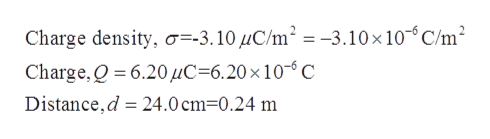# d

Question
49 views

The figure shows a very large nonconducting sheet that has a uniform surface charge density σ = -3.10 μC/m2; it also shows a particle of charge Q = 6.20 μC, at distance d from the sheet. Both are fixed in place. If d = 24.0 cm, at what (a)positive and (b) negative coordinate on the x axis (other than infinity) is the net electric field of the sheet and particle zero? (c) If d = 95.0 cm, at what coordinate is ?

check_circle

Step 1

Given:help_outlineImage TranscriptioncloseCharge density, o=-3.10UC/m2 = -3.10x10-C/m2 Charge,Q 6.20HC 6.20 x 10- C Distance,d 24.0 cm-0.24 m fullscreen
Step 2

Calculation:

According to the given conditions the sheet is negatively charged, and the point charge Q is positively charged.

The magnitude of the electric field of the sheet is,

Step 3

The magnitude of the electric fie...

### Want to see the full answer?

See Solution

#### Want to see this answer and more?

Solutions are written by subject experts who are available 24/7. Questions are typically answered within 1 hour.*

See Solution
*Response times may vary by subject and question.
Tagged in

### Electromagnetic Induction